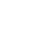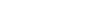# MATLAB Assignment Help Online From Top MATLAB Experts in USA

The Software called MATLAB stands for MATrix LABoratory. It is based on matrices and vectors and has practical application in many areas of science including physics, mathematics and engineering. Every time a student is given a MATLAB task, the range of its uses presents a number of fresh obstacles. Our MATLAB assignment service offers a one-stop shop for all the problems college students encounter when completing their MATLAB assignments.Trustpilot

4.8Home / MATLAB Assignment Help

## Why You Might Need Help With Matlab Assignment

There are several reasons why college students worldwide turn to ‘AssignmentHelpCenter’ for MATLAB assignment assistance. Some of the most common reasons are: Students require help finishing their MATLAB project before the deadline because they are unable to understand the complex MATLAB concepts. The variables, vectors, matrices, images, and structures included in MATLAB assignments are too irritating and perplexing for students. Our MATLAB assignment service is trusted by thousands of students from top universities like Harvard University, MIT, Oxford University, Edinburgh University, University of Cambridge, Stanford University, Princeton University and many others who are pursuing undergraduate and graduate degrees in computer science and information technology. Use our MATLAB assignment support service at the most affordable prices, if you are unsure of your ability to produce an amazing MATLAB assignment.

## Areas Covered by MATLAB Assignments

The following is a list of the main scientific, technical, and statistical fields in which our MATLAB specialists offer online MATLAB assignment assistance:

• Mathematical modeling and computation
• Image processing in MATLAB
• Algorithm creation and synthesis
• Graphical User Interface (GUI) Development
• Data exploration and analysis
• Testing prototypes and simulating

Our programmers are able to comprehend the intricacies of each and every MATLAB programming application, and they can produce your assignment in accordance with the demands of the selected topic. We urge you to use our online MATLAB assignment assistance since we don’t want you to compromise on the caliber of your MATLAB assignments.

## MATLAB Assignment Applications

MATLAB has applications in

• Automated Driving Systems
• Computational Biology
• Control Systems
• Data Science
• Deep Learning
• Electrification
• Embedded Systems
• Enterprise and IT Systems
• FPGA, ASIC, and SoC Development
• image Processing and Computer Vision
• Internet of Things
• Machine Learning
• Mechatronics

## Hire the Best MATLAB Assignment Services and Tutors

AssignmentHelpCenter has some of the best MATLAB assignment experts providing exceptional help with MATLAB assignments and projects. The MATLAB assignment writers hold up to Ph.D. qualification in various areas of MATLAB, like data analysis, signal processing, embedded systems and many more. The MATLAB programmers working with us try to keep the coding simple, yet of high-quality. Every MATLAB project completed by our programmers is written from scratch, and all the codes are well-commented for future references.

The MATLAB assignment writers are aware of the fact that correct documentation can fetch you higher marks. Therefore, they also provide free quality assurance reports along with the MATLAB code. To have a clear idea of the quality we maintain, you can read the free MATLAB assignment samples written by our experts. Place your order as soon as possible and get exceptional quality offered by our highly qualified professionals.

## Traits Of Trusted MATLAB Assignment Writing Companies

Students seek online MATLAB assignment help because they feel it is essential to get a helping hand for their upcoming MATLAB assignment. They think so because each time they seek help with MATLAB assignments, our MATLAB assignment writers ensure to revise their assignments thoroughly before returning.

Furthermore, our MATLAB assignment experts also provide correct in-text citations and a list of references in your desired style guide. Now; let’s take a look at what are the key features of the MATLAB assignment solution.

### Free revisions

Each time you seek online MATLAB assignment help from our MATLAB assignment helpers, they ensure to offer you free revisions.

### Perfect coding

Our programmers code your all assignments perfectly. They are the Masters of MATLAB and can solve the most complex problems.

### Correct citations

Citations are an essential part of coding. Each time you seek a MATLAB assignment solution from our MATLAB assignment helpers, they ensure to put correct citations for each code.

## Why Choose Our MATLAB Assignment Support Service

tudents select AssignmentHelpCenter.Com for a MATLAB assignment solution because we are the only website that always offers 100% authentic papers. Moreover, we are the only MATLAB assignment writing services that have been in the industry for more than a decade. If you are not convinced, read on and find more reasons to seek our online MATLAB assignment help:

### Our MATLAB experts

AssignmentHelpCenter is a trusted MATLAB assignment provider. All our MATLAB assignment helpers have PhDs in their academic domain and many years of experience in MATLAB programming. Many of our MATLAB assignment specialists have worked for leading tech companies.

### Our experience

AssignmentHelpCenter.Com is recognized as the world’s best MATLAB assignment writing service because of the expertise and experience our MATLAB specialists possess. We match you with an appropriate qualified expert to ensure every bit of your requirements is satisfied.

### Our quality of work

The quality of the MATLAB assignments and solutions we create speak for themselves. Each time you resort to our MATLAB assignment support and seek MATLAB assignment experts, we ensure to provide MATLAB assignments and solutions that are 100% authentic and plagiarism-free. Hence you should seek our online MATLAB homework help to We are indeed the best MATLAB assignment service in the entire industry. Therefore, waste no time and resort to us for the desired results

## Why do Students Seek MATLAB Homework Help?

To correctly comprehend the assignment, you must be familiar with theory and mathematical techniques. You also need to understand how to use the supplied data and the best possible solutions to a problem. The method of completing MATLAB homework should integrate theory, maths, data, and a student’s growing field knowledge. However, if there are too many moving components in this process, it might go horribly wrong, causing tension and complete avoidance of the topic. The best-written homework assignments include test cases in compiled function p-files (.p), allowing you to validate your findings. This significantly lowers task uncertainty, but it necessitates more university resources.

Students require assistance when badly written homework, confusing theoretical courses, and personal problems accumulate to a point where they want to just give up. Receiving assistance is crucial, especially when no university professor is available to you at home. For this reason, getting MATLAB assistance online is a great option!

## How to Hire Our MATLAB Programmers

Hiring MATLAB experts and MATLAB programmers from AssignmentHelpCenter is quick and simple.

• Submit order by providing the details (deadline, lecture slides, etc.)
• A writer starts working on your order once you confirm the order.

We always encourage students to complete homework on their own, however, it is obvious that in certain circumstances it is not possible – and so we are always here, ready to help.

## MATLAB Homework Assistance – Example Solution

A MATLAB program can produce three-dimensional graphics using the functions surf and mesh.

`[X,Y] = meshgrid(-10:0.25:10,-10:0.25:10); f = sinc(sqrt((X/pi).^2+(Y/pi).^2)); mesh(X,Y,f); axis([-10 10 -10 10 -0.3 1]) xlabel('{\bfx}') ylabel('{\bfy}') zlabel('{\bfsinc} ({\bfR})') hidden off [X,Y] = meshgrid(-10:0.25:10,-10:0.25:10); f = sinc(sqrt((X/pi).^2+(Y/pi).^2)); surf(X,Y,f); axis([-10 10 -10 10 -0.3 1]) xlabel('{\bfx}') ylabel('{\bfy}') zlabel('{\bfsinc} ({\bfR})') `

Output: